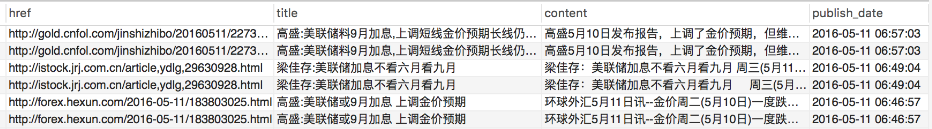gensim文档相似度判断

# 一、基本概念

## TF-IDF

• TF：term frequency，词频

$$词频(TF) = 某个词在文章中的出现次数$$

$$词频(TF) = \frac{某个词在文章中的出现次数}{文章的总次数}$$

$$词频(TF) = \frac{某个词在文章中的出现次数}{该文出现次数最多的词的出现次数}$$

• IDF：inverse document frequency，逆文档频率

$$IDF = log(\frac{语料库的文档总数}{包含该词的文档数+1})$$

• TF-IDF

$$TF-IDF = 词频(TF) \times逆文档频率(IDF)$$

## TF-IDF计算步骤

• 第一步：把每个网页文本分词，称为词包（bag of words）

• 第二步：统计网页（文档）总数M

• 第三步：统计第一个网页次数N，计算第一个网页第一个词在该网页中出现的次数n，再找出该词在所有文档中出现的次数m。

$$\frac{\frac{n}{N}}{\frac{m}{M}}$$

• 第四步：重复第三步，计算出一个网页所有词的tf-idf值。

• 第五步：重复第四步，计算出所有网页每个词的tf-idf值。

## 余弦相似度 (cosine similiarity)

$$cos\theta=\frac{a^2+b^2-c^2}{2ab}$$

# 二、相似度计算步骤

1，处理用户查询

• 第一步：对用户查询进行分词

• 第二步： 根据网页库（文档）的数据， 计算用户查询中每个词的tf-idf值。

2，相似度的计算

# 三、gensim介绍

Gensim是一个相当专业的主题模型Python工具包。是一个用于主题建模、文档索引以及使用大规模语料数据的相似性检索。相比RAM，它能处理更多的输入数据。作者称它是“根据纯文本进行非监督性建模最健壮、最有效的、最让人放心的软件。”

gensim安装： pip install gensim

# 四、实现步骤

## 1，中文分词## 3，建立LSI模型

lsi最核心的意义是将训练文档向量组成的矩阵SVD分解，并做了一个秩为2的近似SVD分解。

# 五、进阶

## Python实现代码

Author: Binger Chen